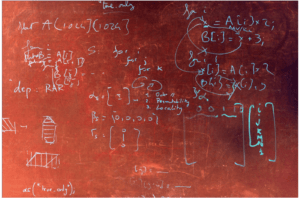## Combinatorial Multigrid for Lattice Quantum ChromodynamicsLattice Quantum Chromodynamics (LQCD) is a numerical approach to understanding the strong nuclear force, in which space and time are discretized into a 4D lattice. Calculating the quark propagator involves inverting sparse, roughly symmetric and diagonally dominant matrices with trillions of entries… Continue reading “Combinatorial Multigrid for Lattice Quantum Chromodynamics”